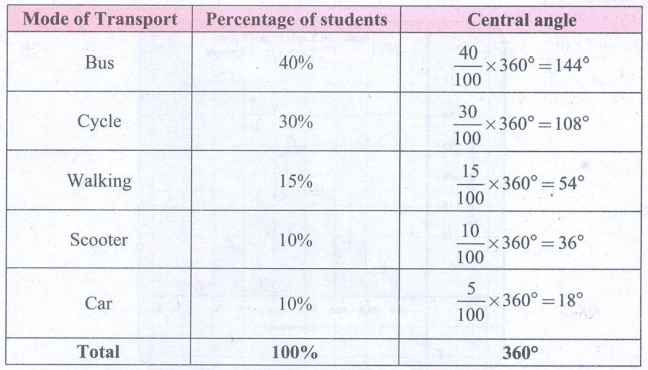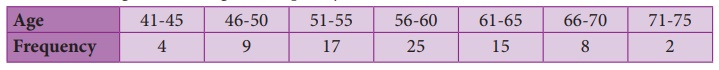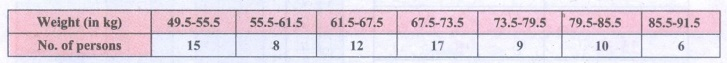Home | | Maths 8th Std | Exercise 6.3

# Exercise 6.3

8th Maths : Chapter 6 : Statistics : Exercise 6.3 : Miscellaneous Practice problems, Challenge Problems, Text Book Back Numerical problems, Exercises Questions with Answers, Solution

Exercise 6.3

Miscellaneous Practice Problems

1. Draw a pie chart for the given table.Solution:
Converting the area in percentage into components parts of 360°, we have.Continental Area.2. The data on modes of transport used by the students to come to school are given below. Draw a pie chart for the data.Solution:

Converting the percentage into components parts of 360°, we haveMode of Transport by students.3. Draw a histogram for the given frequency distribution.Solution:

The given distribution is discontinuous.

Lower boundary = lower limit – 1/2 (gap between the adjacent class interval)

= 41− 1/2 (l) = 40.5

Upper boundary = Upper limit + 1/2 (gap between the adjacent class interval)

= 45 + 1/2(1) = 45.5

Now continuous frequency table is as below4. Draw a histogram and the frequency polygon in the same diagram to represent the following data.Solution:

The given distribution is discontinuous.

Lower boundary = lower limit – 1/2 (gap between the adjacent class interval)

= 50 – 1/2(1) = 49.5

Upper boundary = Upper limit + 1/2 (gap between the adjacent class interval)

= 55 + 1/2(1) = 55.5

The continuous frequency table is as below.Challenging problems

5. Form a continuous frequency distribution table and draw histogram from the following data.Solution:

Converting into continuous distribution we have6. A rupee spent in a cloth manufacturing company is distributed as follows. Represent this in a pie chart.Solution:

1 Rupee = 100 paise.Expenditure of a cloth manufacturing company.7. Draw a histogram for the following data.Solution:

Since mid values are given, the given distributors is discontinuous.

Lower boundary = lower limit – 1/2 (gap between the adjacent class interval)

= 15 – 1/2(10) = 10

Upper boundary = Upper limit + 1/2(gap between the adjacent class interval)

= 15 + 1/2(10) = 20

The continuous distribution will be as follows.Tags : Questions with Answers, Solution | Statistics | Chapter 6 | 8th Maths , 8th Maths : Chapter 6 : Statistics
Study Material, Lecturing Notes, Assignment, Reference, Wiki description explanation, brief detail
8th Maths : Chapter 6 : Statistics : Exercise 6.3 | Questions with Answers, Solution | Statistics | Chapter 6 | 8th Maths